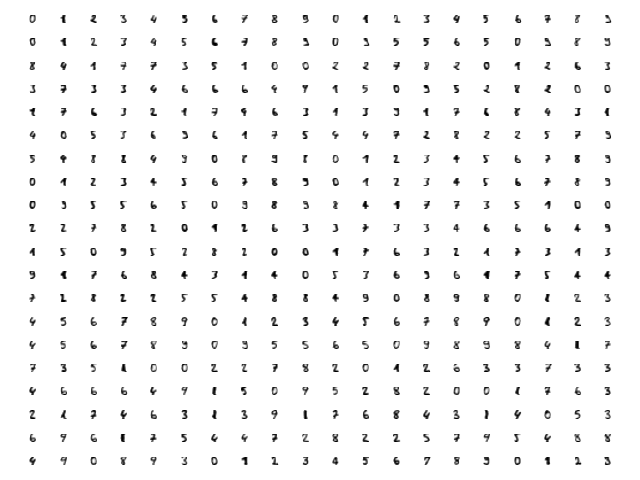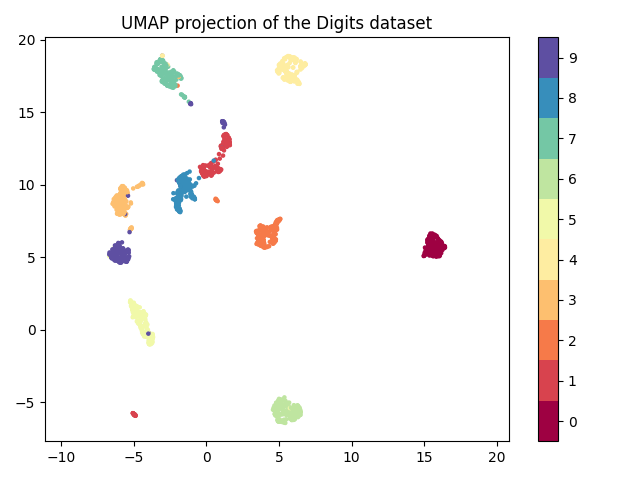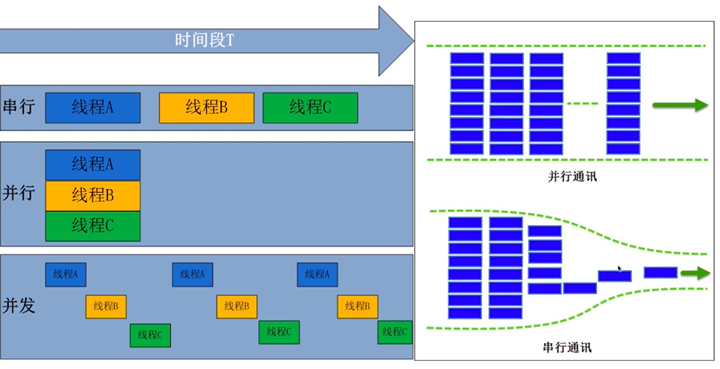# 比t-SNE更好的降维算法UMAP

## UMAP简介

UMAP ，全称uniform manifold approximation and projection，统一流形逼近与投影，是基于黎曼几何和代数拓扑的理论框架结构构建的。在处理大数据集时，UMAP优势明显，运行速度更快，内存占用小。UMAP是一种降维技术，类似于t-SNE，可用于可视化，但也可用于一般的非线性降维。 该算法基于关于数据的三个假设：

• 数据均匀分布在黎曼流形上（Riemannian manifold）
• 黎曼度量是局部恒定的（或可以这样近似）
• 流形是局部连接的

## UMAP的使用

umap包继承了sklearn类，因此与其他具有相同调用API的sklearn转换器紧密地放在一起。UMAP主要参数

• n_neighbors：这决定了流形结构局部逼近中相邻点的个数。更大的值将导致更多的全局结构被保留，而失去了详细的局部结构。一般来说，这个参数应该在5到50之间，10到15是一个合理的默认值。
• min_dist: 这控制了嵌入的紧密程度，允许压缩点在一起。数值越大，嵌入点分布越均匀;数值越小，算法对局部结构的优化越精确。合理的值在001到0.5之间，0.1是合理的默认值。
• n_components：作为许多scikit学习降维算法的标准，UMAP提供了一个n_components参数选项，允许用户确定将数据嵌入的降维空间的维数。与其他一些可视化算法（如t-SNE）不同，UMAP在嵌入维度上具有很好的伸缩性，因此您可以使用它进行二维或三维的可视化。
• metric: 这决定了在输入空间中用来测量距离的度量的选择。已经编写了各种各样的度量标准，用户定义的函数只要经过numba的JITd处理就可以传递。

from sklearn.datasets import load_digits
import matplotlib.pyplot as plt

fig, ax_array = plt.subplots(20, 20)
axes = ax_array.flatten()
for i, ax in enumerate(axes):
ax.imshow(digits.images[i], cmap='gray_r')
plt.setp(axes, xticks=[], yticks=[], frame_on=False)
plt.show()


Digits Data每个数字是64维的向量，先查看数据：from sklearn.datasets import load_digits
import matplotlib.pyplot as plt
import umap
import numpy as np

reducer = umap.UMAP(random_state=42)
embedding = reducer.fit_transform(digits.data)
print(embedding.shape)

plt.scatter(embedding[:, 0], embedding[:, 1], c=digits.target, cmap='Spectral', s=5)
plt.gca().set_aspect('equal', 'datalim')
plt.colorbar(boundaries=np.arange(11) - 0.5).set_ticks(np.arange(10))
plt.title('UMAP projection of the Digits dataset')
plt.show()##### 如何理解Python装饰器##### Python协程与异步